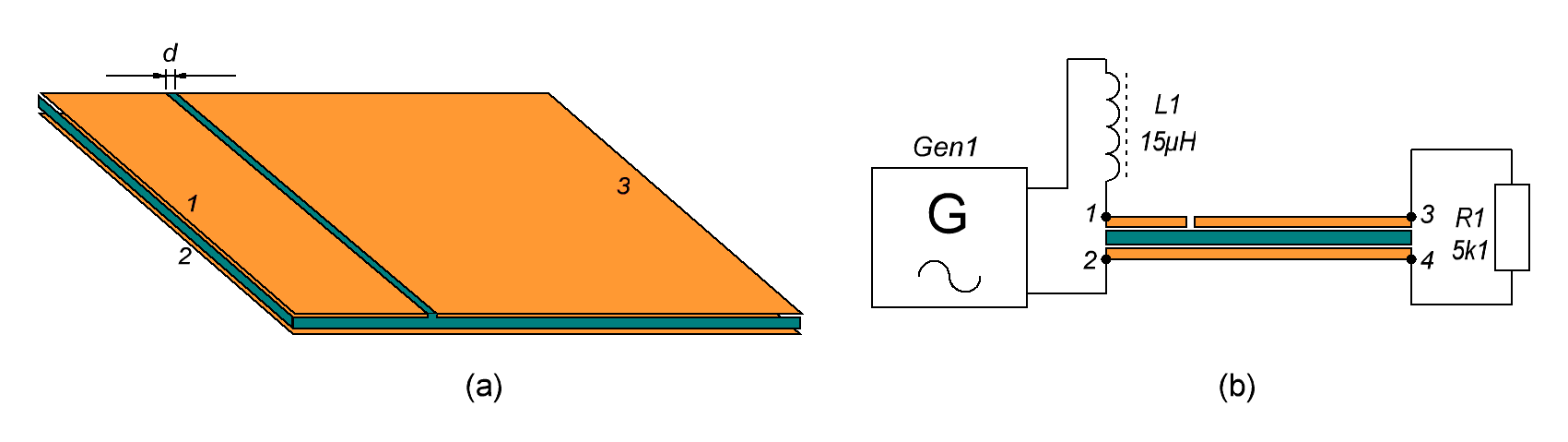Research website of Vyacheslav Gorchilin
2020-12-06
T-shaped capacitor property
This experiment is devoted to the study of the claimed property, and the corresponding formula, in . We are talking about the use of the reactive component of power for the transfer of energy, which in its essence implies such transfer by displacement currents . One way to test this possibility is to study the transfer of energy in a T-shaped capacitor (Fig. 1a) from one plate (1) to another (3). In this case, its bottom plate is common (2) is also a common wire in the circuit (Fig. 1b).
Practically, for such a capacitor, a double-sided fiberglass with dimensions of 180*130 mm and a thickness of 2.5 mm was chosen, which originally consisted of two thin copper plates and an insulator between them. In figure (1), we will depict copper plates in orange, and the dielectric in green. On the top copper plate, a 2 mm thick d cut was made, which electrically began to divide the top plate into two (1) and (3), in a ratio of about 1 to 4. Between the plates (1-2), through a choke L1 with a low throughput capacity, a Gen1 sinusoidal oscillator was connected, and the active resistance R1 was connected to the plates (3-4), from which the output signal was taken. It should be noted that the entire circuit under study was brought into resonance at a frequency that in this design was 3.4 MHz.Fig.1. T-shaped capacitor, 1a - appearance, 1b - connection diagram
Further, an equivalent circuit of this experiment was drawn up for the case when the T-shaped capacitor would consist of separate capacities C1-C3 (Fig. 2). Using the MicroCap11 simulator, the frequency response for the input and output of such a circuit was obtained. I must say that this simulator quite accurately reflects real processes and if the circuit were assembled in this way, then we would get exactly the same results. Figure (3) shows the frequency response (the dependence of the signal amplitude on frequency) at points (1-2) in the red graph, and in the blue graph - in points (3-4). These graphs reflect the actual dependencies of the `` disassembled '' T-capacitor. But it turned out that a single-board capacitor (Fig. 1) has slightly different properties, which we reflected in the green graph (Fig. 3).Fig.2. Equivalent schemaFig.3. Frequency response of the input and output of the T-capacitor and its equivalent
A distinctive feature of a single-board T-capacitor turned out to be that after a certain minimum frequency, there is almost no drop in the amplitude at the output (3-4), up to a decrease in frequency to 10 kHz. In this case, if the T-capacitor would consist of separate capacities (as in Fig. 2), the drop in amplitude would be approximately proportional to the decrease in frequency. Thus, at a frequency of 10 kHz, the ratio of the output amplitudes of the single board to the disassembled The T-capacitor was approximately 12 times!
Also, another experiment was carried out, in which another copper plate was installed on top of the T-capacitor, through an insulator, completely covering it with its area and connected to a common wire. It turned out that the plates (1) and (3) were in the middle of an artificially created waveguide. In this case, the picture was about the same, except for the amplitude peak at the output of the circuit: it was slightly less, which resulted in an even flatter frequency response (Fig. 3, gray graph).
Conclusions
As a result of the experiment, it was possible to confirm the possibility of transferring energy using displacement currents, and hence using the reactive component of power. This conclusion can be made on the basis of the data obtained and the distinctive feature of the T-shaped capacitor, which follows from its frequency response. At such low frequencies, about 12 kHz, with small overall dimensions of the circuit, wave processes cannot occur, therefore, the transfer of additional energy is possible only due to the bias currents, which are formed in any capacitor when the amplitude of the voltage on its plates changes.
Thus, for such a transmission method, it is necessary: a conventional waveguide, which can be any dielectric medium, exciting a resonant circuit at its input and an energy receiver at its output.
Materials used
1. D.S. Strebkov, A.I. Nekrasov. Resonant methods of transmission of electrical energy, p. 3.5 (3.2)
2. Wikipedia. Current offsets